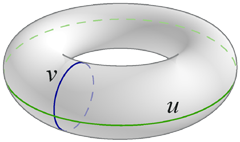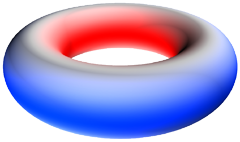]> The Curvature of the Torus

# The Curvature of the Torus

Let's get bent

We begin with the parameterized surface:$x u v = x = c + a cos v cos u y = c + a cos v sin u z = a sin v$

Take the partial derivatives of this parameterization and compute inner products to find the coefficients of the first fundamental form: $E = c + a cos v 2 , F = 0 , G = a 2$ . This gives us the line element $ds 2 = c + a cos v 2 du 2 + a 2 dv 2$ , from which we read off the metric:

$g ij = c + a cos v 2 0 0 a 2$

Some straightforward and boring computation yields the nonzero Christoffel symbols of the second kind:

$Γ uv u = Γ vu u = − a sin v c + a cos v Γ uu v = 1 a sin v c + a cos v$

Another two pages of index juggling and basic algebra gives the nonzero components of the Riemann tensor:

$R vuv u = − R vvu u = a cos v c + a cos v R uvu v = − R uuv v = 1 a cos v c + a cos v$

Contract to get the Ricci tensor:

$R ij = R imj m = 1 a cos v c + a cos v 0 0 a cos v c + a cos v$

Finally, contract with the upper form of the metric to get the Ricci scalar (a.k.a. the curvature scalar):

$R = g ij R ij = 2 cos v a c + a cos v$

The result is twice the Gaussian curvature, as expected.

What does the Gaussian curvature tell us about the torus? Since c > a the denominator is always positive, so the sign of the curvature is determined only by cos v. The illustration shows regions of different curvature: on the outside of the torus curvature is positive (blue), on the inside it's negative (red), and at the top and bottom circles it's zero (grey).Understanding the torus's curvature will help us in our search for the torus's geodesics.

Last updated 25 April 2005
http://www.rdrop.com/~half/math/torus/curvature.xhtml
All contents released into the public domain by Mark L. Irons